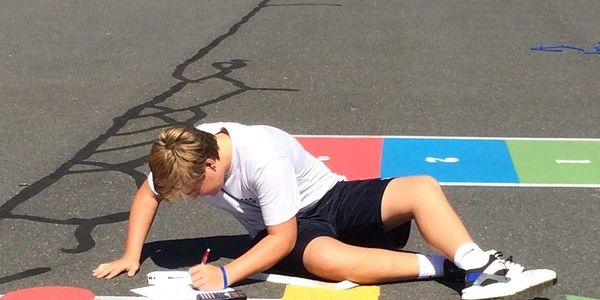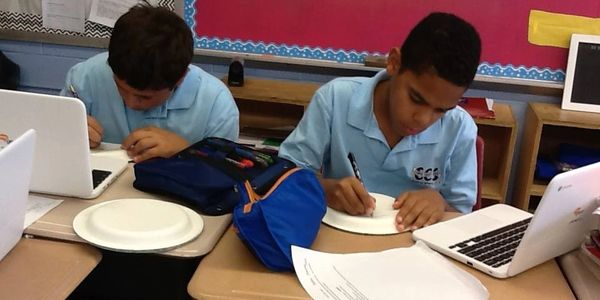The vision statement of the Catholic schools of the Archdiocese of Hartford challenges its schools to graduate students who are productive moral citizens and critical thinkers. The discipline of mathematics is key to the achievement of that vision. Students, at Corpus Christi, learn to observe, predict, analyze, and solve problems related to routine daily tasks.Significant moral decisions require the techniques of problem solving learned in a strong mathematics curriculum. By its nature, mathematics promotes logical and abstract thinking. The methodical approach needed to reach conclusions fosters the self-discipline necessary to solve simple and complex exercises. Knowledge of mathematical processes and skills are the tools needed to solve problems and construct valid arguments in other disciplines. Mathematics serves as a tool in both the natural and social sciences and stands as a logical foundation for the consideration of moral and ethical issues by Catholic Christian thinkers.

Mathematical instruction at Corpus Christi School encompasses the following instructional programs that will enable students to be proficient in the following:

• Students understand numbers, ways of representing numbers, relationships among numbers, and number systems
• Students understand meanings of operations and how they relate to one another
• Students compute fluently and make reasonable estimates
• Students understand patterns, relations, and functions
• Students represent and analyze mathematical situations and structures using algebraic symbols
• Students use mathematical models to represent and understand quantitative relationships
• Students analyze change in various contexts
• Students analyze characteristics and properties of two- and three-dimensional geometric shapes and develop mathematical arguments about geometric relationships
• Students specify locations and describe spatial relationships using coordinate geometry and other representational systems
• Students apply transformations and use symmetry to analyze mathematical situations
• Students use visualization, spatial reasoning, and geometric modeling to solve problems
• Students understand measurable attributes of objects and the units, systems, and processes of measurement
• Students apply appropriate techniques, tools, and formulas to determine measurements
• Students formulate questions that can be addressed with data and collect, organize, and display relevant data to answer them
• Students select and use appropriate statistical methods to analyze data
• Students will use their study of math to make data-driven moral decisions and to promote justice in the worldSignificant moral decisions require the techniques of problem solving learned in a strong mathematics curriculum. By its nature, mathematics promotes logical and abstract thinking. The methodical approach needed to reach conclusions fosters the self-discipline necessary to solve simple and complex exercises. Knowledge of mathematical processes and skills are the tools needed to solve problems and construct valid arguments in other disciplines. Mathematics serves as a tool in both the natural and social sciences and stands as a logical foundation for the consideration of moral and ethical issues by Catholic Christian thinkers.